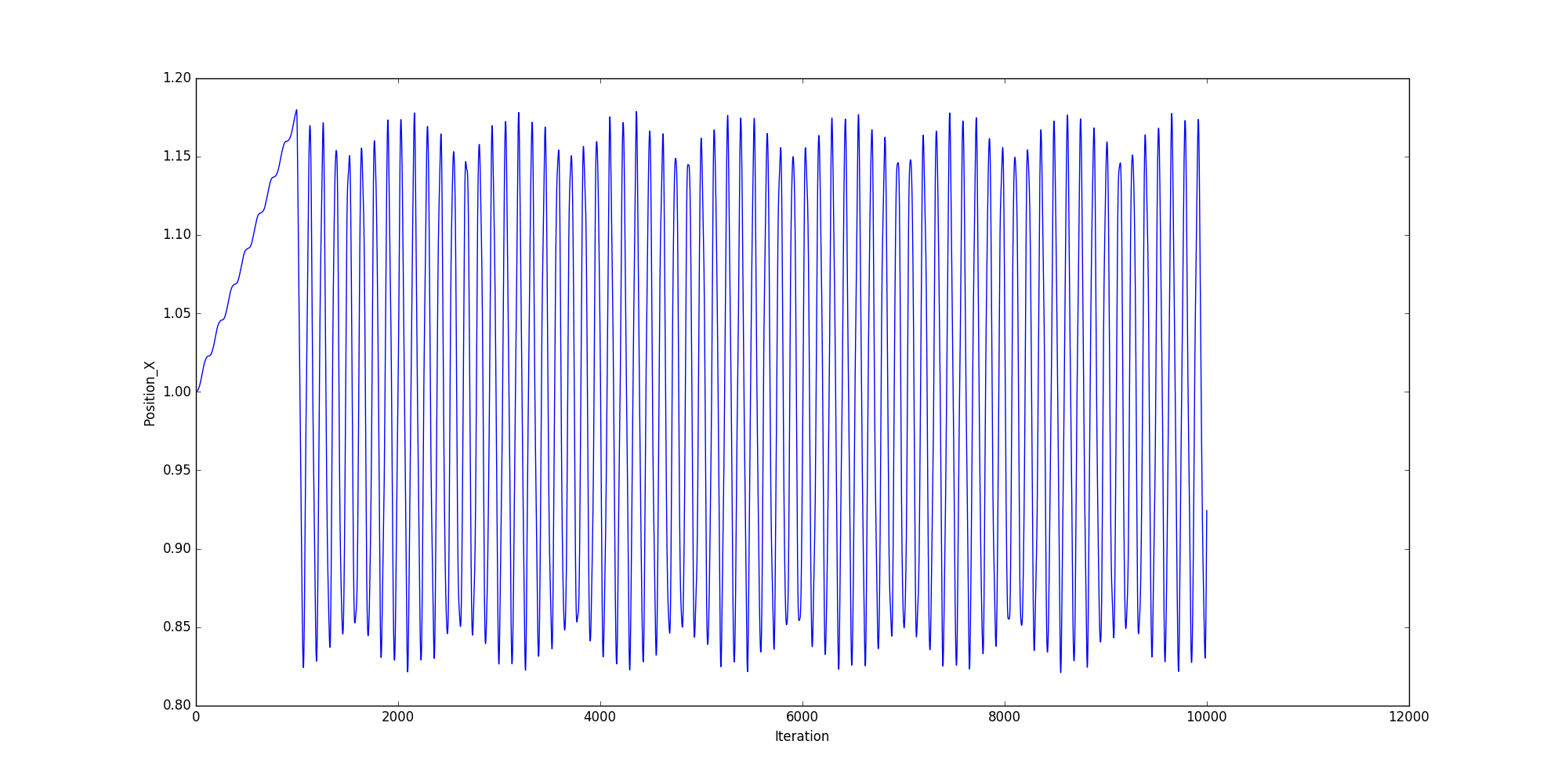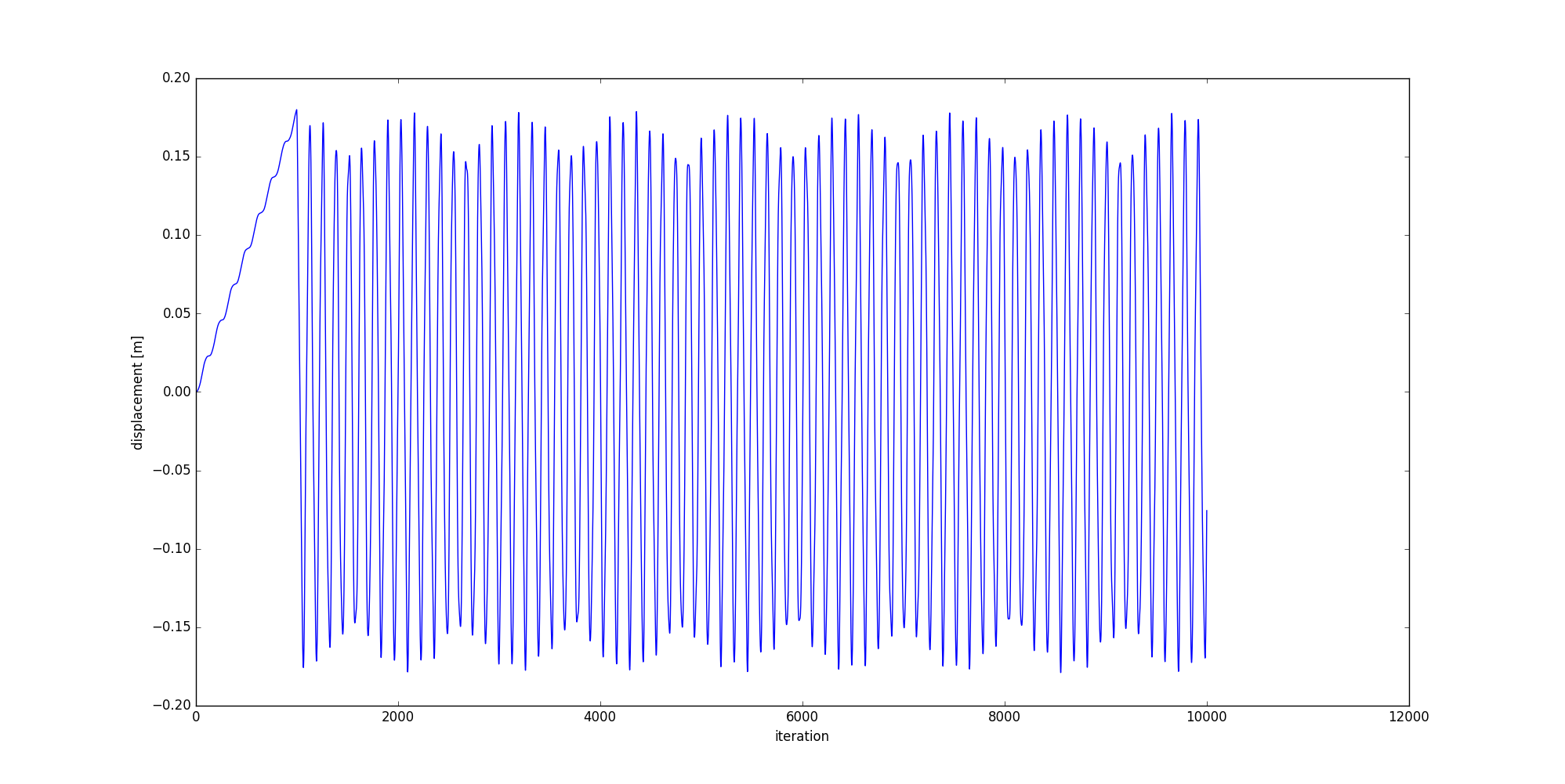# Tutorials

basic

The numerical sensors are provided by the `libUtil` library. They allow to monitor any numerical values and write them in a separated file. Using the sensors requires two steps :

• initializing sensors. It consists in “placing” the numerical sensors to monitor the required values.

• triggering sensors acquisition with the `_WriteSensorData` standard plugin that collects sensor values and writes them in a file during the processing loop.

# Prerequisites

You must follow the previous tutorial step before starting this tutorial. You need the simple tensile test built in the previous tutorial step.

# Goal

It is proposed here to use sensors for monitoring the iteration number and the displacement of the “left” discrete element set.

# Initializing sensor

To initialize sensors, a new plugin will be used. As it was previously shown, you can use the `granoo3-project` tool to build a new plugin from scratch.

The `PlugIn_InitSensor.hpp` file must look like

And the `PlugIn_InitSensor.hpp` file must look like

The `PlugIn_InitSensor.py` file must look like

Adding a sensor is easy. You just need to invoke the `granoo.sensor.add` static method that takes a method or a function as first argument and a string label as second argument.

In this example, only the `run()` `run(self)` method of the plugin is used. Two numerical sensors were created. The first one monitors the iteration number and the second one monitors the position of the discrete element placed at left. Numerical sensors are built with the static method that takes : an object, a pointer to a class method of this object and a string used to labeled the sensor. Be aware, never use temporary object here ! Because the object must be alive when the sensors is triggered with the `WRITE-SENSOR-DATA` plugin.

To avoid any further problems, do not use any special characters or white space in sensor labels.

# Building and running

In order to execute the `InitSensor` plugin, you must add the following line in your `tensile.inp` file at the end of the `PreProcessing` section.

In addition, you must add the following line at the end of the `Processing` section.

Now, you can compile and run your simulation

Now, you can run your simulation

If you take a look at the Output directory, it must contain a `Sensors.txt`. This file must look like

There is a lot of software solutions to plot this kind of file : spreadsheet softwares or scripting solutions such as gnuplot or matplotlib. Among these solutions, GranOO embeds a useful python script that allows easy plotting of the Sensors.txt files.

This script is named `granoo3-plot`. It uses the well known python matplotlib library. You can display a help message with the `-h` option as follows. The following command plots the position along X versus iteration number.

It gives the following chart# Post-treating the “Sensors.txt” file

The previous section has plotted the position of the “right” discrete element along the X axis versus the iteration number. Now, we want to plot the displacement along the X axis. To do that, we need to post-treat the Sensors.txt file. To compute the displacement, the initial value of the position, given by the sensor, must be subtracted to each measured position values.

The `granoo3-plot` script can be used also as a python module. This module embeds useful functions that help users to post-treat and to plot a `Sensors.txt` file. The following python script named `Plot.py` shows how to :

• import the `granoo3-plot` script file as a module,
• parse the `Sensors.txt` file,
• store the read values as numpy arrays,
• do mathematic operations on these arrays and
• plot the result with matplotlib.

Now, you can create a new python file named `plot.py` that contains

If you run this script with the following command

It gives the following chart